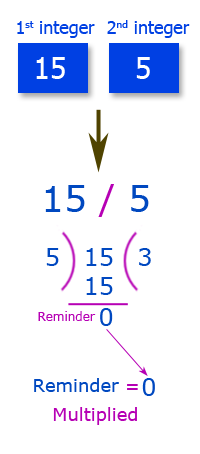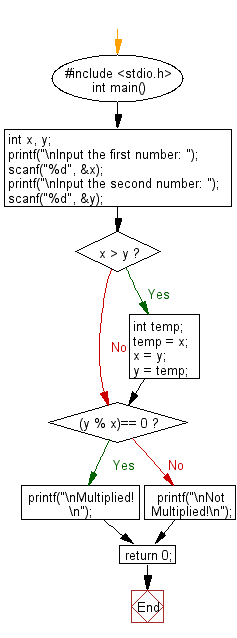﻿ C Program: Checks whether two integers have been multiplied# C Exercises: Reads two integers and checks whether they are multiplied or not

## C Basic Declarations and Expressions: Exercise-24 with Solution

Write a C program that reads two integers and checks whether they are multiplied or not.

Pictorial Presentation:C Code:

``````#include <stdio.h>

int main() {
int  x, y;
printf("\nInput the first number: ");
scanf("%d", &x);
printf("\nInput the second number: ");
scanf("%d", &y);

if(x > y)
{
int temp;
temp = x;
x = y;
y = temp;
}

if((y % x)== 0)
{
printf("\nMultiplied!\n");
}
else
{
printf("\nNot Multiplied!\n");
}

return 0;
}
``````

Sample Output:

```Input the first number: 5

Input the second number: 15

Multiplied!
```

Flowchart:C Programming Code Editor:

What is the difficulty level of this exercise?

Test your Programming skills with w3resource's quiz.

﻿

## C Programming: Tips of the Day

Is there a way to specify how many characters of a string to print out using printf()?

The basic way is:

`printf ("Here are the first 8 chars: %.8s\n", "A string that is more than 8 chars");`

The other, often more useful, way is:

`printf ("Here are the first %d chars: %.*s\n", 8, 8, "A string that is more than 8 chars");`

Here, you specify the length as an int argument to printf(), which treats the '*' in the format as a request to get the length from an argument.

You can also use the notation:

```printf ("Here are the first 8 chars: %*.*s\n",
8, 8, "A string that is more than 8 chars");```

This is also analogous to the "%8.8s" notation, but again allows you to specify the minimum and maximum lengths at runtime - more realistically in a scenario like:

`printf("Data: %*.*s Other info: %d\n", minlen, maxlen, string, info);`

The POSIX specification for printf() defines these mechanism

Ref : https://bit.ly/3u32GyO